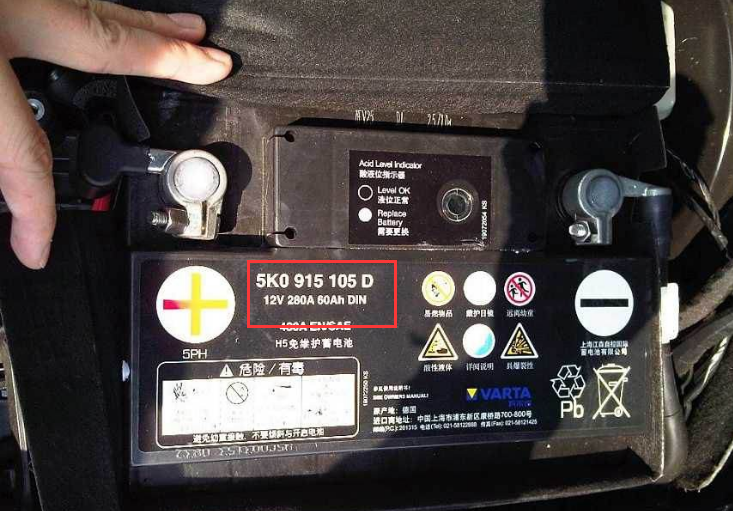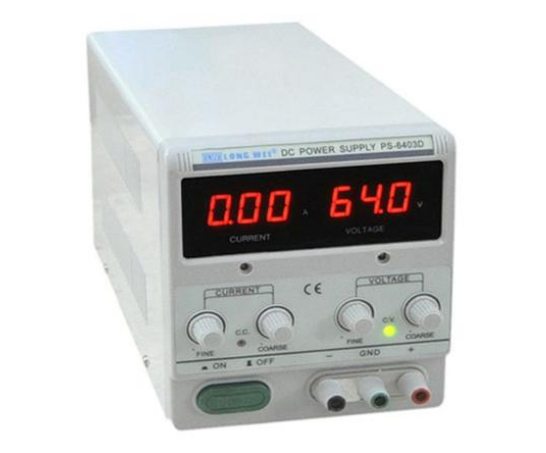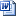# How to calculate and test MDVR power consumption

2020-04-10    702 views

First, different vehicles have different voltage. So the power consumption is different.Such as the above picture, this vehicle has 60Ah.

The cpacity marked on the battery: 60AH, 70AH, 80AH, 100AH, etc. refers to the battery's ability to store electricity. The larger the data, the more electricity it can store and the more resistant it is to discharge The longer you use the same amount of electricity

Take the 60AH battery as an example: 60AH means:

1. 60 amp hours, referred to as 60 amp hours. It means that if our electrical appliance uses one ampere discharge, then it can be used for 60 hours, if it uses two amps, it can be used for 30 hours, and 10 amps, It can be used for 6 hours. If you use 20 amps to discharge, the battery can be used for 3 hours. Assuming that we use 60 amps to discharge, then it can only be used for one hour. How many ampere hours represents the storage of this battery. ability.

2. So when the MDVR works, the ampere is more than 1, and 12V can support MDVR boot and run, you can use adjustable power meter to test the ampere and then you will get an approximate value that how long the battery of the vehicle will support for the MDVR running.3. If need figure specific consumption, you can use the vehicle to test this point.How to calculate and test MDVR power consumption.doc